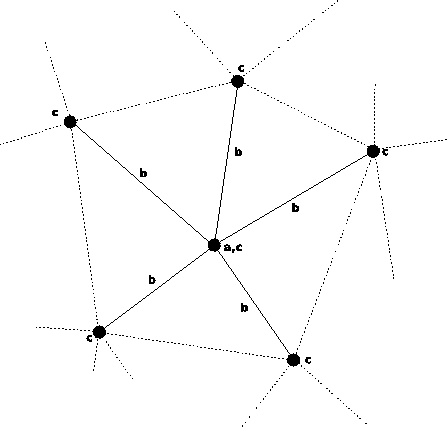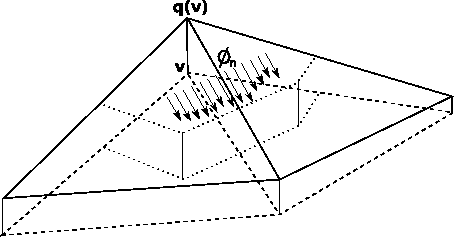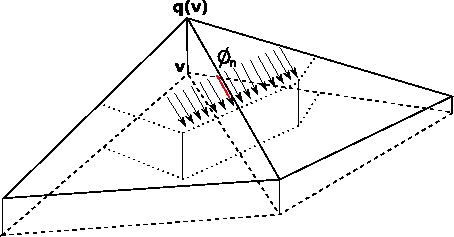## 3. 2. 3 Topological Structure of the Finite Volume Scheme

The finite volume scheme shown in Section 3.2.1 requires the following topological operations: from a vertex all incident cells are required (determination of the integration domain) and for these cells all incident vertices have to be traversed (determination of the function values). Such a traversal scheme is identical to the traversal scheme of finite elements which only have vertex-based shape functions.The method described in Section 3.2.2 requires all incident edges of the initial vertex for the determination of the integration domain. This integration domain is covered by the union of two cells which are incident with the edge. In order to determine the shape functions given on these cells, the function coefficients associated with the incident vertices of the cell have to be determined.If further requirements of Section 3.2.2 are given or assumed, the evaluation of the finite volume differential operator is obtained by traversing all incident edges of the vertex and subsequently traversing the vertices on the edge for calculating the function value (on the edge only).In the following sections, the second method of Section 3.2.2 is used for the specification of the finite volume schemes. For the discretization of the Laplace equation the requirements for this method are given for the discretization of the semiconductor transport equations  the requirements are assumed to be fulfilled approximately.

Michael 2008-01-16